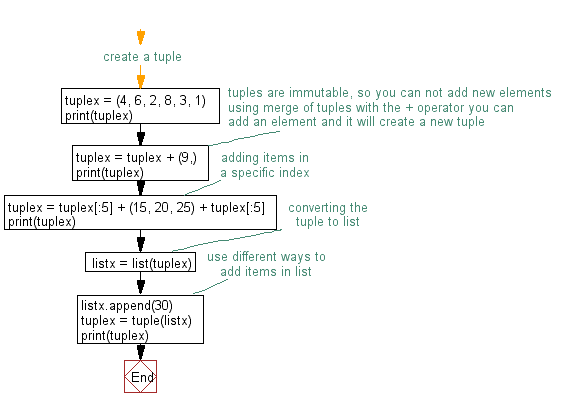﻿ Python: Add an item in a tuple - w3resource# Python Exercise: Add an item in a tuple

## Python tuple: Exercise-5 with Solution

Write a Python program to add an item in a tuple.

Sample Solution:-

Python Code:

``````#create a tuple
tuplex = (4, 6, 2, 8, 3, 1)
print(tuplex)
#tuples are immutable, so you can not add new elements
#using merge of tuples with the + operator you can add an element and it will create a new tuple
tuplex = tuplex + (9,)
print(tuplex)
#adding items in a specific index
tuplex = tuplex[:5] + (15, 20, 25) + tuplex[:5]
print(tuplex)
#converting the tuple to list
listx = list(tuplex)
#use different ways to add items in list
listx.append(30)
tuplex = tuple(listx)
print(tuplex)
```
```

Sample Output:

```(4, 6, 2, 8, 3, 1)
(4, 6, 2, 8, 3, 1, 9)
(4, 6, 2, 8, 3, 15, 20, 25, 4, 6, 2, 8, 3)
(4, 6, 2, 8, 3, 15, 20, 25, 4, 6, 2, 8, 3, 30)
```

Flowchart:## Visualize Python code execution:

The following tool visualize what the computer is doing step-by-step as it executes the said program:

Python Code Editor:

Have another way to solve this solution? Contribute your code (and comments) through Disqus.

What is the difficulty level of this exercise?

Test your Python skills with w3resource's quiz

﻿

## Python: Tips of the Day

Returns the maximum value of a list, after mapping each element to a value using the provided function

Example:

```def tips_max(lst, fn):
return max(map(fn, lst))

print(tips_max([{ 'n': 4 }, { 'n': 2 }, { 'n': 8 }, { 'n': 6 }], lambda v : v['n']))
```

Output:

```8
```